Download Presentation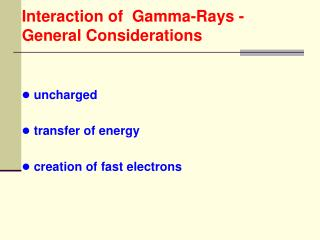Interaction of Gamma-Rays - General Considerations

# Interaction of Gamma-Rays - General Considerations - PowerPoint PPT Presentation

Interaction of Gamma-Rays - General Considerations. uncharged transfer of energy creation of fast electrons. Interaction of Gamma-Rays - Types. photoelectric Compton pair production photodisintegration. Photoelectric Effect.I am the owner, or an agent authorized to act on behalf of the owner, of the copyrighted work described.
Download Presentation## Interaction of Gamma-Rays - General Considerations

An Image/Link below is provided (as is) to download presentation

Download Policy: Content on the Website is provided to you AS IS for your information and personal use and may not be sold / licensed / shared on other websites without getting consent from its author.While downloading, if for some reason you are not able to download a presentation, the publisher may have deleted the file from their server.

- - - - - - - - - - - - - - - - - - - - - - - - - - E N D - - - - - - - - - - - - - - - - - - - - - - - - - -
Presentation Transcript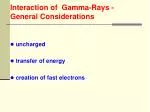Interaction of Gamma-Rays - General Considerations
• uncharged
• transfer of energy
• creation of fast electrons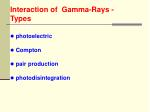Interaction of Gamma-Rays - Types
• photoelectric
• Compton
• pair production
• photodisintegration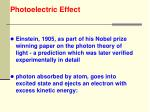Photoelectric Effect
• Einstein, 1905, as part of his Nobel prize winning paper on the photon theory of light - a prediction which was later verified experimentally in detail
• photon absorbed by atom, goes into excited state and ejects an electron with excess kinetic energy: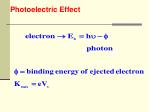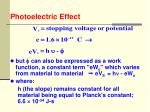Photoelectric Effect
• but  can also be expressed as a work function, a constant term "eWo" which varies from material to material  eVS = h - eWo
• where:
• h (the slope) remains constant for all material being equal to Planck's constant; 6.6  10-34 J-s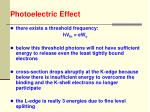Photoelectric Effect
• there exists a threshold frequency:

hVth = eWo

• below this threshold photons will not have sufficient energy to release even the least tightly bound electrons
• cross-section drops abruptly at the K-edge because below there is insufficient energy to overcome the binding and the K-shell electrons no longer participate
• the L-edge is really 3 energies due to fine level splitting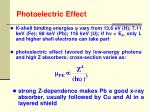Photoelectric Effect
• K-shell binding energies  vary from 13.6 eV (H); 7.11 keV (Fe); 88 keV (Pb); 116 keV (U); if hν < Ek, only L and higher shell electrons can take part
• photoelectric effect favored by low-energy photons and high Z absorbers; cross-section varies as:
• strong Z-dependence makes Pb a good x-ray absorber, usually followed by Cu and Al in a layered shield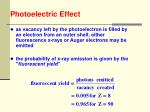Photoelectric Effect
• as vacancy left by the photoelectron is filled by an electron from an outer shell, either fluorescence x-rays or Auger electrons may be emitted
• the probability of x-ray emission is given by the "fluorescent yield"Compton Scattering
• wave interpretation predicts that when electromagnetic radiation is scattered from a charged particle, the scattered radiation will have the same frequency as the incident radiation in all directions
• the scattering of electromagnetic radiation from a charged particle is viewed as a perfectly elastic billiard ballCompton Scattering

γ

4 unknowns: E1,, K, 

3 equations: momentum conservation (2),

energy conservationCompton Scattering
• K = (m - mo)c2 difference between the total energy E of the moving particle and the rest energy Eo (at rest)
• must treat electron relativisticallyCompton Scattering
• while for photons
• energy

K =(p0 – p1)cCompton Scattering
• substitute these expressions for K1, p2 into relativistic electron energy expression to get (after manipulation):
• where Δ  is the shift that the scattered photon undergoes
• the wavelength is usually measured in multiples of "Compton units", the ratio of:Compton Scattering
• wavelength
• the difference in energy Eo - E1 = K is the kinetic energy of the electronCompton Scattering
• the min. electron energy corresponds to min. scattered photon energy (θ = 180º) so that
• this energy Kmax is called the Compton edge
• another form for this equation which uses photon energies instead of wavelengths is:Compton Scattering
• at high incident energies Eo the back scattered photon approaches a constant energyCompton Scattering

 0.511 MeV θ = 90º

 0.255 MeV θ = 180º

• in this limit we find that 0«  ≈ , so that the energy:Compton ScatteringProblem

In a Compton experiment an electron attains kinetic energy of 0.100 MeV when an x-ray of energy 0.500 MeV strikes it. Determine the wavelength of the scattered photon if the electron is initially at restPair Production
• in the process of pair production the energy carried by a photon is completely converted into matter, resulting in the creation of an electron-positron pair

σpp ~ Z2

• since the charge of the system was initially zero, 2 oppositely charged particles must be produced in order to conserve chargePair Production
• in order to produce a pair, the incident photon must have an energy of the pair; any excess energy of the photon appears as kinetic energy of the particlesPair Production
• pair production cannot occur in empty space
• the nucleus carries away an appreciable amount of the incident photon's momentum, but because of its large mass, its recoil kinetic energy, k ≈ p2/2mo, is usually negligible compared to kinetic energies of the electron-positron pair
• thus, energy (but not momentum) conservation may be applied with the heavy nucleus ignored, yielding:

h = m+c2 + m-c2 = k+ + k- + 2moc2

• since the positron and the electron have the same rest mass;

mo = 9.11 x 10-31 kgAnnihilation
• the inverse of pair production can also occur
• in pair annihilation a positron-electron pair is annihilated, resulting in the creation of 2 (or more) photons as shown
• at least 2 photons must be produced in order to conserve energy and momentumAnnihilation
• in contrast to pair production, pair annihilation can take place in empty space and both energy and momentum principles are applicable, so that:
• where k l is the propagation vector:

2(k) = 2/Annihilation

problem:

• how many positrons can a 200 MeV photon produce?
• the energy needed to create an electron-positron pair at rest is twice the rest energy of an electron, or 1.022 MeV; therefore maximum number of positrons equals:Photodisintegration
• absorber nucleus captures a -ray and in most instances emits a neutron:

9Be( ,n) 8Be

• important for high energy photons from electron accelerators
• cross-sections are « total cross-sectionsCombined Effects
• total attenuation coefficient 
• in computing shielding design the above equation is used
• this is the fraction of the energy in a beam that is removed per unit distance of absorberCombined Effects
• the fraction of the beam's energy that is deposited in the absorber considers only the energy transferred by the photoelectron, Compton electron, and the electron pair
• energy carried away by the scattered photon by Compton and by annihilation is not included ExponentialAbsorption
• due to the different interaction of -rays with matter, the attenuation is different than with α or  particles
• intensity of a beam of photons will be reduced as it passes through material because they will be removed or scattered by some combination of photoelectric effect, Compton scattering and pair production
• reduction obeys the exponential attenuation law:Exponential Absorption

I = Ioe-t

• where:

I0 = -ray intensity at zero absorber

thickness

t = absorber thickness

I = -ray intensity transmitted

 = attenuation coefficient

• if the absorber thickness is measure in cm, then  is called linear attenuation coefficient (l) having dimensions "per cm"Exponential Absorption

• if the absorber thickness "t" is measured in g/cm2, then (m) is called mass attenuation coefficient (m) having dimensions cm2/g

where  is the density of the absorberExponential Absorption
• what percent of an incident x-ray passes through a 5 mm material whose linear absorption is 0.07 mm-1?Exponential Absorption
• a monochromatic beam of photons is incident on an absorbing material
• if the intensity is reduced by a factor of 2 by 8 mm of material, what is the absorption coefficient?Exponential Absorption

Half-Value Thickness (HVT)

• thickness of absorber which reduces the intensity of a photon beam to 1/2 its incident value
• find HVT of aluminum if  = 0.070 mm-1Exponential Absorption

Atomic Attenuation Coefficient a

• fraction of an incident -ray beam that is attenuated by a single atom, or the probability that an absorber atom will interact with one of the photons
• where a is referred to as a cross-section and has the units barns(0.435 cm-1)

Exponential Absorption
• what is the thickness of Al and Pb to transmit 10% of a 0.1 MeV -ray?Exponential Absorption
• if we have a 1.0 MeV  - ray:
• compute the density thickness at 0.1 MeVExponential Absorption
• at 1.0 MeV
• this shows that Pb is only slightly better on a mass basis than Al
• however for low energy photons Pb is much better
• in general, for energies between 0.8  5 MeV almost all materials, on a mass basis, have approximately the same -ray attenuating propertiesPhoton Interactions - Problem

• 1-MeV photons are normally incident on a 1-cm lead slab
• the mass attenuation coefficient of lead (density = 11.35 g/cm3) is 0.0708 cm2/g and the atomic weight is 207.2Photon Interactions - Problem
• calculate the linear attenuation coefficient
• what fraction of 1-MeV photons interact in a 1-cm lead slab?
• what thickness of lead is required for half the incident photons to interact?
• calculate the mean free pathPhoton Interactions - Problem

Solution

a. the linear attenuation coefficient  is obtained by multiplying the mass attenuation coefficient by the density

 = (0.0708 cm2 g-1)  (11.35 g cm-3 = 0.804 cm-1.

b. if Io photons are incident on the lead slab and I photons penetrate it without interacting, then the fraction not interacting is given by:

I/Io = exp[-  x]

= exp[(-0.804 cm-1)(1 cm)] = 0.448

the fraction of photons interacting is then: 

1 - 0.448 = 0.552Photon Interactions - Problem
• substituting I/Io = 1/2 in eq. (1) above and rearranging yields:

x = ln2/μ = 0.693/0.804 = 0.862 cmPhoton Interactions - Problem
• the mean free path (MFP) is the average distance that an incident photon travels before interaction and is the reciprocal of the attenuation coefficient:

MFP = 1/μ = 1/0.804 = 1.24 cm

• this is also numerically equal to the "relaxation length“
• the relaxation length is the shield thickness needed to attenuate a narrow beam of monoenergetic photons to 1/e (= 0.368) of its original intensity and can be derived by substituting I/Io = 1/e